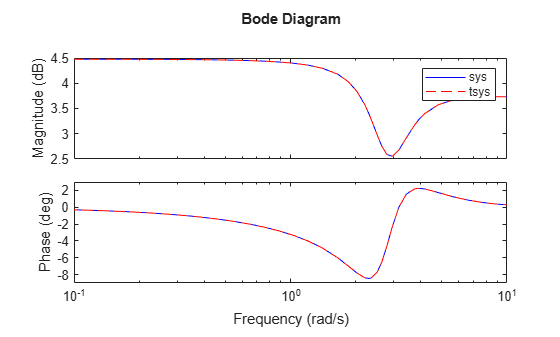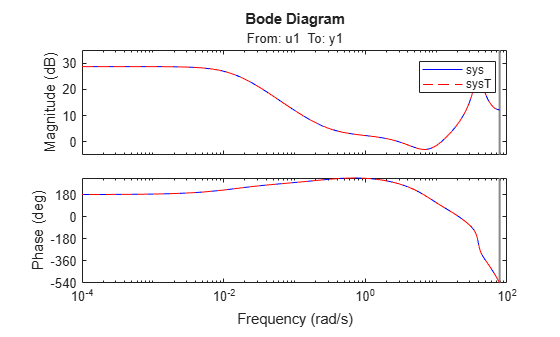# ss2ss

State coordinate transformation for state-space model

## Syntax

``sysT = ss2ss(sys,T)``

## Description

`ss2ss` performs the similarity transformation z = Tx on the state vector x of a state-space model. For more information, see Algorithms.

example

````sysT = ss2ss(sys,T)` performs the state-coordinate transformation of `sys` using the specified transformation matrix `T`. The matrix `T` must be invertible.```

## Examples

collapse all

Perform a similarity transform for a state space model.

Generate a random state-space model and a transformation matrix.

```rng(0) sys = rss(5); t = randn(5);```

Perform the transformation and plot the frequency response of both models.

```tsys = ss2ss(sys,t); bode(sys,'b',tsys,'r--') legend```The responses of both models match closely.

`ss2ss` applies state transformation only to the state vectors of the numeric portion of the generalized model.

Create a `genss` model.

`sys = rss(2,2,2) * tunableSS('a',2,2,3) + tunableGain('b',2,3)`
```sys = Generalized continuous-time state-space model with 2 outputs, 3 inputs, 4 states, and the following blocks: a: Tunable 2x3 state-space model, 2 states, 1 occurrences. b: Tunable 2x3 gain, 1 occurrences. Type "ss(sys)" to see the current value, "get(sys)" to see all properties, and "sys.Blocks" to interact with the blocks. ```

Specify a transformation matrix and obtain the transformation.

```T = [1 -2;3 5]; tsys = ss2ss(sys,T)```
```tsys = Generalized continuous-time state-space model with 2 outputs, 3 inputs, 4 states, and the following blocks: a: Tunable 2x3 state-space model, 2 states, 1 occurrences. b: Tunable 2x3 gain, 1 occurrences. Type "ss(tsys)" to see the current value, "get(tsys)" to see all properties, and "tsys.Blocks" to interact with the blocks. ```

Decompose both models.

```[H,B,~,~] = getLFTModel(sys); [H1,B1,~,~] = getLFTModel(tsys);```

Obtain the transformation separately on the model from decomposed `sys`.

`H2 = ss2ss(H,T);`

Compare this transformed model with the model from decomposed `tsys`.

`isequal(H1,H2)`
```ans = logical 1 ```

Both models are equal.

The file `icEngine.mat` contains one data set with 1500 input-output samples collected at the a sampling rate of 0.04 seconds. The input `u(t)` is the voltage (V) controlling the By-Pass Idle Air Valve (BPAV), and the output `y(t)` is the engine speed (RPM/100).

Use the data in `icEngine.mat` to create a state-space model with identifiable parameters.

```load icEngine.mat z = iddata(y,u,0.04); sys = n4sid(z,4,'InputDelay',2);```

Specify a random transformation matrix.

`T = randn(4);`

Obtain the transformation.

`sysT = ss2ss(sys,T);`

Compare the frequency responses.

```bode(sys,'b',sysT,'r--') legend```The responses match closely.

`ss2ss` also lets you perform similarity transformation for models with complex coefficients.

For this example, generate a random state-space model with complex coefficients.

```rng(0) sys = ss(randn(5)+1i*randn(5),randn(5,3),randn(2,5)+1i*randn(2,5),0,.1);```

Specify a transformation matrix containing complex data.

`T = randn(5)+1i*randn(5);`

Obtain the transformation.

`sysT = ss2ss(sys,T);`

Compare the singular values of the frequency response.

```sigma(sys,'b',sysT,'r--') legend```The responses match closely for both branches.

## Input Arguments

collapse all

Dynamic system, specified as a SISO, or MIMO dynamic system model. Dynamic systems that you can use include:

If `sys` is an array of state-space models, `ss2ss` applies the transformation `T` to each individual model in the array.

Transformation matrix, specified as an n-by-n matrix, where n is the number of states. `T` is the transformation between the state vector of the state-space model `sys` and the state vector of the transformed model `sysT`. (See Algorithms.)

## Output Arguments

collapse all

Transformed state-space model, returned as a dynamic system model of the same type as `sys`.

## Algorithms

`ss2ss` performs the similarity transformation $\overline{x}=Tx$ on the state vector x of a state-space model.

This table summarizes the transformations returned by `ss2ss` for each model form.

Input ModelTransformed Model

Explicit state-space models of the form:

`$\begin{array}{l}\stackrel{˙}{x}=Ax+Bu\\ y=Cx+Du\end{array}$`
`$\begin{array}{l}\stackrel{˙}{\overline{x}}=TA{T}^{-1}\overline{x}+TBu\\ y=C{T}^{-1}\overline{x}+Du\end{array}$`

Descriptor (implicit) state-space models for the form:

`$\begin{array}{c}E\stackrel{˙}{x}=Ax+Bu\\ y=Cx+Du\end{array}$`
`$\begin{array}{c}E{T}^{-1}\stackrel{˙}{\overline{x}}=A{T}^{-1}\overline{x}+Bu\\ y=C{T}^{-1}\overline{x}+Du\end{array}$`

Identified state-space (`idss`) models of the form:

`$\begin{array}{c}\frac{dx}{dt}=Ax+Bu+Ke\\ y=Cx+Du+e\end{array}$`
`$\begin{array}{l}\stackrel{˙}{\overline{x}}=TA{T}^{-}{}^{1}\overline{x}+TBu+TKe\\ y=C{T}^{-}{}^{1}\overline{x}+Du+e\end{array}$`

## Compatibility Considerations

expand all

Behavior changed in R2021b

Errors starting in R2021b

(Control System Toolbox) | (Control System Toolbox) |

### Topics

Introduced before R2006a

## Support#### Try MATLAB, Simulink, and Other Products

Get trial now